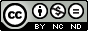Disentangling the marginal problem in quantum chemistry

Benavides Riveros, Carlos
Gracia Bondía, José Mariano (dir.)

Abstract: It is well known that determining the energy of molecules and other quantum many-body systems reduces in the standard approximation to optimizing a simple linear functional of a 12-variable object, the two-electron reduced density matrix (2-RDM). The difficulty is that the variation ensemble for that functional has never been satisfactorily determined. This is known as the N-representability problem of quantum chemistry (which to a large extent is a problem of quantum information theory). The situation has given rise to competing research programs, typically trading more complicated functionals for simpler representability conditions. Chief among them is density functional theory, based on a three-variable object for which the N-representability is trivial, whereas the exact functional is very strange indeed, and probably forever unknowable. An intermediate position is occupied by 1-RDM functional theory. Postulated by Pauli to explain the electronic structure of atoms and molecules, the exclusion principle establishes an upper bound of 1 for the fermionic natural occupation numbers, accordingly allowing no more than one electron in each quantum state. This is a necessary and sufficient condition for a 1-RDM to be the contraction of an ensemble N-body density matrix. The fermionic one-body quantum marginal problem asks whether given natural occupation numbers can arise from an antisymmetric (ensemble or pure) N-particle state. The configuration interaction method affords optimal descriptions of quantum states of atoms and molecules by expanding the wave function in terms of orbital-based configurations of Slater determinants. For these systems, the dimension of the Hilbert space grows binomially with m, the number of spin-orbitals in the basis of the one-particle Hilbert space, and N, the number of electrons of the system. It has been observed that for the rank-six approximation of a pure-state N=3 system, the occupation numbers satisfy some additional constraints, stronger than the Pauli exclusion principle. The recent analysis by Alexander Klyachko and coworkers of the one-body marginal problem of the pure N-fermion state establishes a systematic approach to this type of constraints. In fact, for a pure quantum system of N electrons arranged in m spin-orbitals the occupation numbers satisfy a set of linear inequalities, known as generalized Pauli constraints (GPC). These inequalities define a convex polytope of allowed states in R^m. They are conditions for a 1-RDM to be the contraction of a N-body state. When one of the GPC is completely saturated, the system is said to be pinned, and it lies on one of the facets of the polytope. The nature of those conditions has been explored till now only in a few systems: a model of three spinless fermions confined to a one-dimensional harmonic potential, the lithium isoelectronic series and ground and excited states of some three- and four-electron molecules for the rank being at least twice the number of electrons. For all these systems the inequalities are (quite often) nearly saturated. This is the so-called quasipinning phenomenon. In this PhD thesis we have taken up the challenge of using numerical and analytical methods to examine pinned and quasipinned states, for atoms and molecules, starting from scratch with configuration-interaction and multiconfiguration self-consistent methods. This procedure serves to study the occurrence of quasipinning in realistic systems. A second goal is to show how the subsets of pinned states defined by GPC give rise to the most efficient approach from a computational viewpoint, yielding the leading order of the electron-electron correlations. As a consequence, we underline in this thesis the theoretical and practical importance of Klyachko's approach to the quantum marginal problem and its impact on the competing research programs to determine feasible electronic densities, 1-RDM, 2-RDM, intracular distributions or Wigner density quasiprobabilities. In relation with the above, our research provides a new variational optimization method for few-fermion ground states. We quantitatively confirm its high accuracy for quasipinned systems and derive an upper bound on the error of the correlation energy given by the ratio of the numerical value of the Klyachko inequality and the distance to the Hartree-Fock point. Depending on the details of the algorithm, we are able to reach 98%-99% of the correlation energy for such systems.

Pal. clave: física teórica ; química física

Knowledge area: Física teórica

Department: Física Teórica

Nota: Tesis-Univ. Zaragoza, Física Teórica, 2015